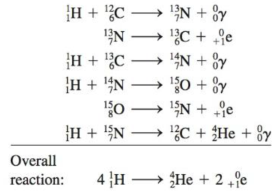Chapter 19, Problem 83CP

Chapter
Section
Textbook Problem

# In addition to the process described in the text, a second process called the carbon-nitrogen cycle occurs in the sun:a. What is the catalyst in this process?b. What nucleons are intermediates?c. How much energy is released per mole of hydrogen nuclei in the overall reaction? (The atomic masses of H 1 1 and H 2 4 e are 1.00782 u and 4.00260 u, respectively.)

(a)

Interpretation Introduction

Interpretation: A carbon nitrogen cycle is given. The catalyst used in the process, nucleons intermediates and the energy released per mole of hydrogen nuclei is to be stated.

Concept introduction: The difference between the sum of masses of the component nucleons and the actual mass of a nucleus is known as the mass defect and it can be used to calculate the nuclear binding energy.

To determine: The catalyst involved in the process.

Explanation

The catalyst is 612C .

The 612

(b)

Interpretation Introduction

Interpretation: A carbon nitrogen cycle is given. The catalyst used in the process, nucleons intermediates and the energy released per mole of hydrogen nuclei is to be stated.

Concept introduction: The difference between the sum of masses of the component nucleons and the actual mass of a nucleus is known as the mass defect and it can be used to calculate the nuclear binding energy.

To determine: The nucleons intermediates involved in the cycle.

(c)

Interpretation Introduction

Interpretation: A carbon nitrogen cycle is given. The catalyst used in the process, nucleons intermediates and the energy released per mole of hydrogen nuclei is to be stated.

Concept introduction: The difference between the sum of masses of the component nucleons and the actual mass of a nucleus is known as the mass defect and it can be used to calculate the nuclear binding energy.

### Still sussing out bartleby?

Check out a sample textbook solution.

See a sample solution

#### The Solution to Your Study Problems

Bartleby provides explanations to thousands of textbook problems written by our experts, many with advanced degrees!

Get Started